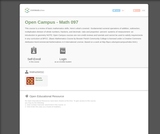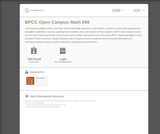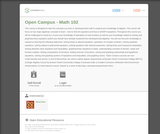# 3 Results

View
Selected filters:
• Bossier Parish Community CollegeOnly Sharing Permitted
CC BY-NC-ND
Rating

This course is a review of basic mathematics skills. Here's what's covered:
-fundamental numeral operations of addition, subtraction, multiplication
division of whole numbers, fractions, and decimals
-ratio and proportion
-percent
-systems of measurement
-an introduction to geometry
NOTE: Open Campus courses are non-credit reviews and tutorials and cannot be used to satisfy requirements in any curriculum at BPCC. (Basic Mathematics Course by Bossier Parish Community College is licensed under a Creative Commons Attribution-NonCommercial-NoDerivatives 4.0 International License.
Based on a work at http://bpcc.edu/opencampus/index.html.)

Subject:
Mathematics
Material Type:
Full Course
Provider:
Bossier Parish Community College
Author:
Michelle Barnickel
07/05/2018Only Sharing Permitted
CC BY-NC-ND
Rating

In this beginning algebra course, you'll learn about fundamental operations on real numbers, exponents, solving linear equations and inequalities, applications, functions, graphing linear equations, slope, and systems of linear equations. This course was created by Bossier Parish Community College as part of its MOOC series "Open Campus." NOTE: Open Campus courses are non-credit reviews and tutorials and cannot be used to satisfy requirements in any curriculum at BPCC. (Beginning Algebra Course by Bossier Parish Community College is licensed under a Creative Commons Attribution-NonCommercial-NoDerivatives 4.0 International License. Based on a work at http://bpcc.edu/opencampus/index.html.)

Subject:
Mathematics
Material Type:
Assessment
Full Course
Lecture
Lecture Notes
Provider:
Bossier Parish Community College
Author:
Gail Hendrix
07/05/2018Only Sharing Permitted
CC BY-NC-ND
Rating

This course is designed to take the concepts you learn in developmental math to expand your knowledge of algebra. This course will focus on two major algebraic concepts to learn - how to SOLVE equations and how to GRAPH equations. Throughout this course you will be challenged to recall ALL of your prior knowledge of operations of real numbers as well as your knowledge related to solving and graphing linear equations (which you should have already mastered from developmental algebra). You will use this prior knowledge to expand on learning the following objectives: solving linear & rational equations. operations of complex numbers, solving quadratic equations, solving radical & polynomial equations, solving equations with rational exponents, solving linear and compound inequalities, solving absolute value equations and inequalities, graphing linear equations & slope, understanding concepts of domain, range and function notation, finding compositions of functions, finding inverses of functions, solving and graphing exponential and logarithmic equations, solving and graphing systems of equations and inequalities, and graphing conics.

*Open Campus courses are non-credit tutorials and cannot, in and of themselves, be used to satisfy degree requirements at Bossier Parish Community College (BPCC). (College Algebra Course by Bossier Parish Community College is licensed under a Creative Commons Attribution-NonCommercial-NoDerivatives 4.0 International License. Based on a work at http://bpcc.edu/opencampus/index.html.)

Subject:
Mathematics
Material Type:
Full Course
Provider:
Bossier Parish Community College
Author:
Stacey Black